Miscellaneous

Chapter 9 Class 11 Straight Lines
Serial order wise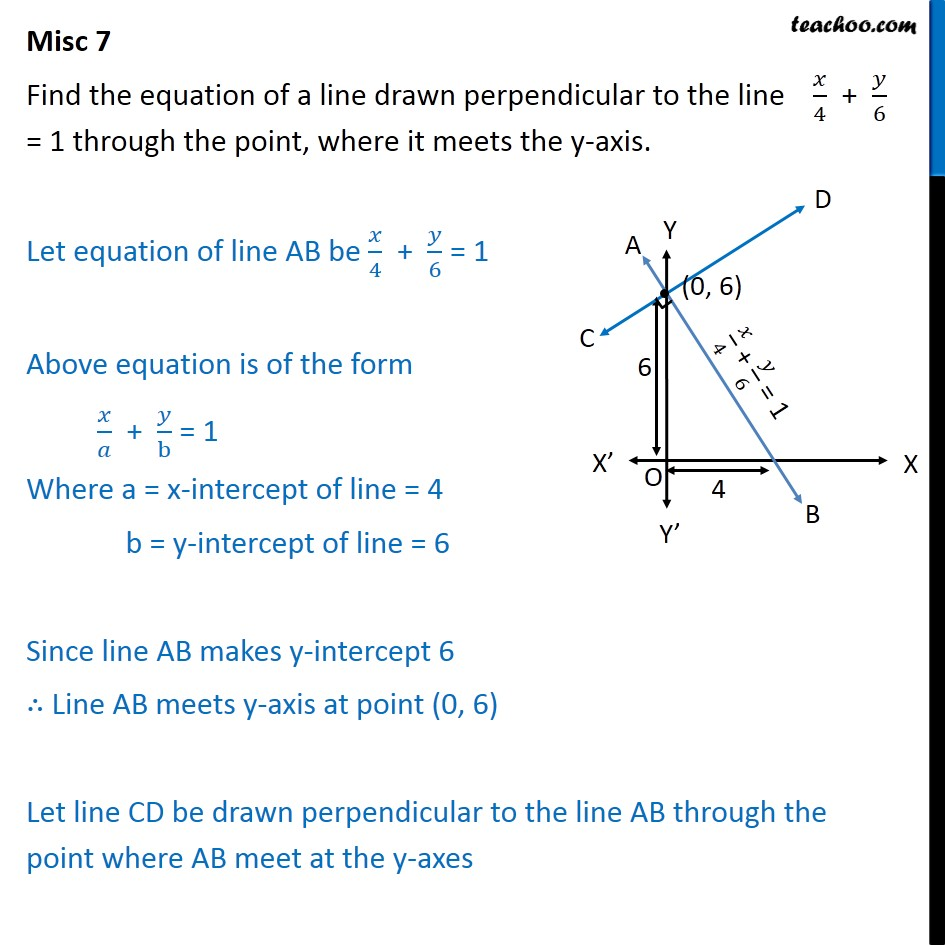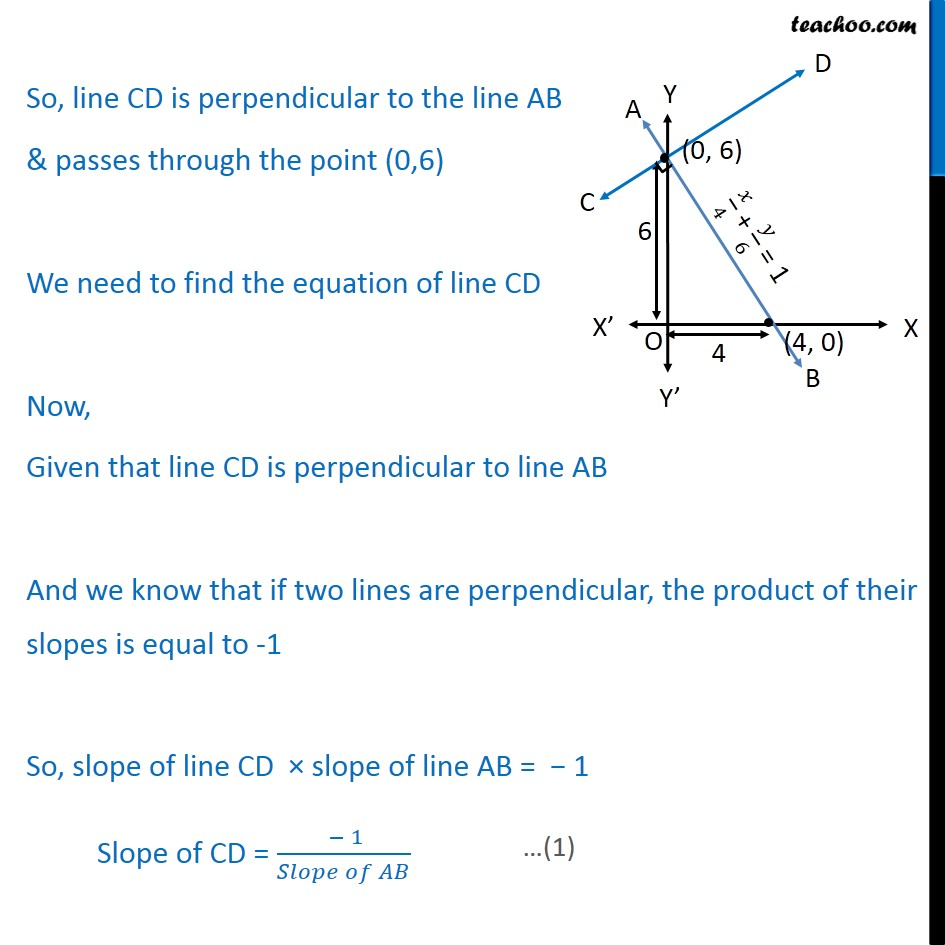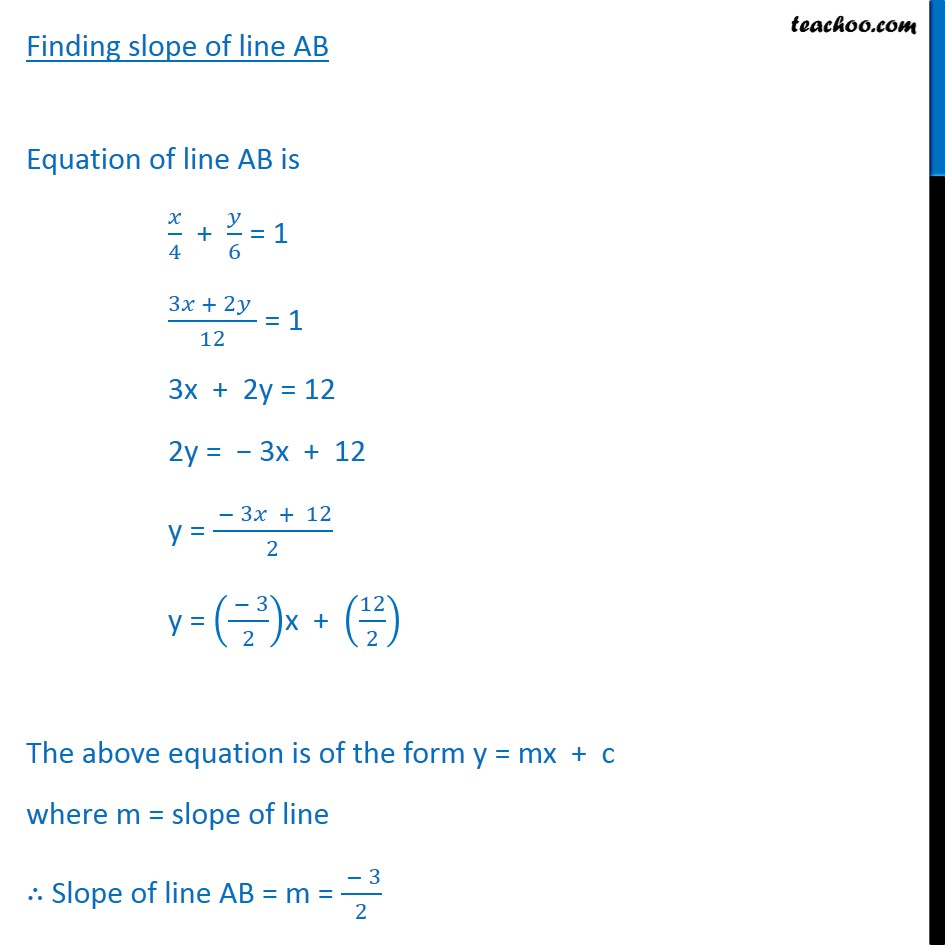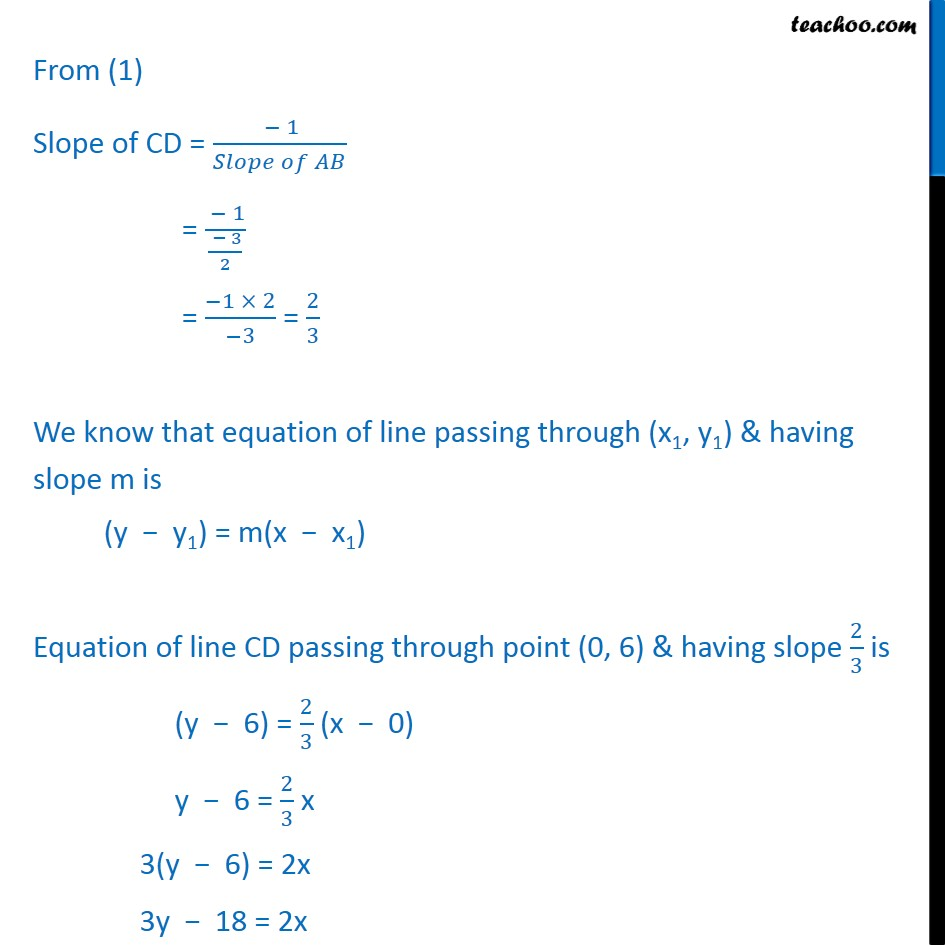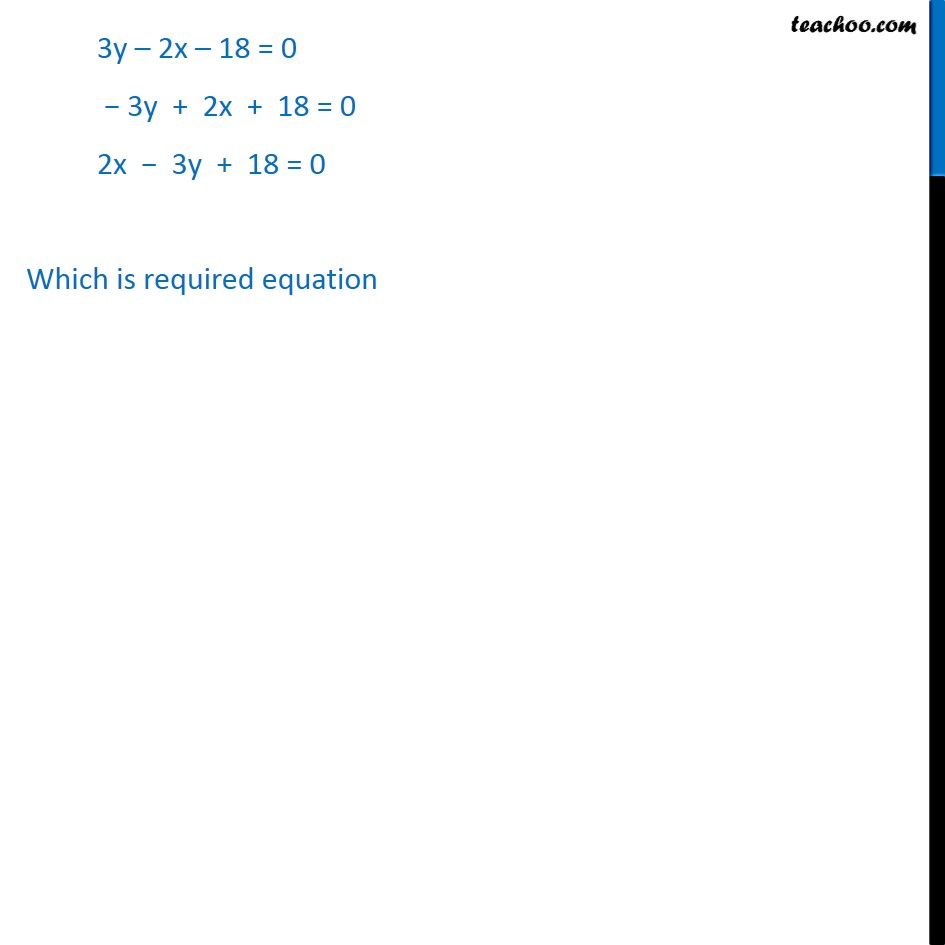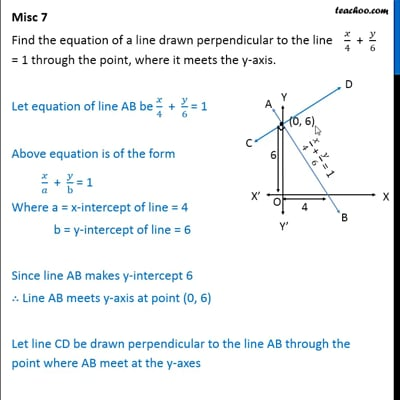This video is only available for Teachoo black users

Learn in your speed, with individual attention - Teachoo Maths 1-on-1 Class

### Transcript

Misc 6 Find the equation of a line drawn perpendicular to the line 𝑥/4 + 𝑦/6 = 1 through the point, where it meets the y-axis. Let equation of line AB be 𝑥/4 + 𝑦/6 = 1 Above equation is of the form 𝑥/𝑎 + 𝑦/b = 1 Where a = x-intercept of line = 4 b = y-intercept of line = 6 Since line AB makes y-intercept 6 ∴ Line AB meets y-axis at point (0, 6) Let line CD be drawn perpendicular to the line AB through the point where AB meet at the y-axes So, line CD is perpendicular to the line AB & passes through the point (0,6) We need to find the equation of line CD Now, Given that line CD is perpendicular to line AB And we know that if two lines are perpendicular, the product of their slopes is equal to -1 So, slope of line CD × slope of line AB = − 1 Slope of CD = ( − 1)/(𝑆𝑙𝑜𝑝𝑒 𝑜𝑓 𝐴𝐵) Finding slope of line AB Equation of line AB is 𝑥/4 + 𝑦/6 = 1 (3𝑥 + 2𝑦 )/12 = 1 3x + 2y = 12 2y = − 3x + 12 y = ( − 3𝑥 + 12)/2 y = (( − 3)/2)x + (12/2) The above equation is of the form y = mx + c where m = slope of line ∴ Slope of line AB = m = ( − 3)/2 From (1) Slope of CD = ( − 1)/(𝑆𝑙𝑜𝑝𝑒 𝑜𝑓 𝐴𝐵) = ( − 1)/(( − 3)/2) = (−1 × 2)/(−3) = 2/3 We know that equation of line passing through (x1, y1) & having slope m is (y − y1) = m(x − x1) Equation of line CD passing through point (0, 6) & having slope 2/3 is (y − 6) = 2/3 (x − 0) y − 6 = 2/3 x 3(y − 6) = 2x 3y − 18 = 2x 3y – 2x – 18 = 0 − 3y + 2x + 18 = 0 2x − 3y + 18 = 0 Which is required equation